# 11 Working Between The Types of Grade

Click play on the following audio player to listen along as you read this section.

Now that we’ve established the three ways we can express grade why don’t we start to work between them.We’ll start with what I think is the easiest and that’s ratio and percent. We actually covered it a little in the last section but let’s go through a more thorough explanation.

# Ratio to Percent and Back

Going from a ratio to a percent is straight forward when we have the ratio as a number over 100 like 2 over 100.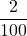As percent means “per one hundred” we end up with 2 percent or 2 out of one hundred. That’s quick and easy.

The problem comes in when we have something not out of 100 such as five over eighty seven.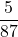Now I can imagine a few of the tradespeople out there might be saying to themselves at this point “We would never end up working with a ratio such as this.” and you would be right. This is not a typical trades number to work with but go ahead and use it just for the purpose of math.

Let’s go through the two steps needed to do this.

Step 1: Change the ratio (or fraction) to a decimal.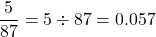Later when we are calculating the total fall on an object using percent the number 0.057 would actually be the number we would work with in the formula. If we want to see what the percent is then we would still have to do  the next step.

Step 2: Change the decimal into a percent.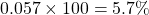By multiplying it by 100 we are basically going back to “per one hundred” and that gives us an answer of 5.7%

Now let’s go the other way and go from a percent back to a ratio.The easiest way to do this might just be to go backwards and start with 5.7% and turn it into a ratio.

Step 1: Change the percent into a decimal.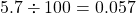Step 2: Change the decimal into a ratio. We know from up above that the ratio is going end up being over 87 so we can work to that.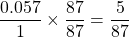First of all the number 0.057 can be written as 0.057 over 1. This gets us into a format which is easy to work with and can visually help us understand what is going on.

We then take both parts of the ratio (or fraction if you will) and multiply them by 87. This ensures that we get a number over 87. What we end up with is what we started with in the beginning.Wait a minute! The only way we knew that it was going to be a ratio out of 87 is because that is what we started with. What if we didn’t know that? What would we do?

Basically we would bring that ratio back to a number over one hundred. And in the end we would end up with 5.7 over 100.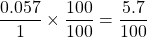Not a nice round number like the first one but still the correct answer. Let’s move on.

# Inches Per Foot to Ratio and Back

This is where things get a little more challenging as changing inches per foot to a ratio involves a couple different units. We have inches, we have feet and then we end up with no units in the ratio. So how do we do this? The first thing we should think about if we are to end up with no units there must be a spot where the units are mathematically cancelled out. Think about that as we go through an example.

Let’s start with one of the more common grades and that is one quarter inch per foot.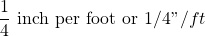Go back to the statement above which stated we should think about how to mathematically cancel out the units to get to no units.Do you see the problem here? How do we cancel when the units are different? We have inches and feet. The solution lies in the idea that we have to change both units into the same thing. This means we have to change both to either inches or both to feet. For our purposes we’ll switch them to inches.

Step 1: Change both inches and feet into inches and put them in a ratio.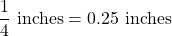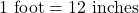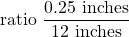Step 2: At this point we have a ratio but does that ratio really work for us all that well? Personally I’d like to see the ratio out of 50 or even better yet out of 100.How do we get the ratio to be out of 100? The answer lies in getting the 12 inches to 100. We do this by figuring out how many times 12 goes into 100 and then we multiply both parts of the ratio by that number. Check out the math below to see how that works.

Start with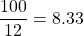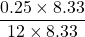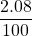In the end a grade of one quarter of an inch per foot gives us a grade of two point zero eight over one hundred as a ratio. Now let’s go backwards.Let’s go from a ratio back to inches per foot. We’ll start with a grade of one over 50.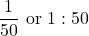What do we do now? At this point I want you to just think for a few seconds about how you would approach this. Even as I’m sitting here writing this part of the book I’m talking to myself and asking myself that question.

Me: Hey self, what am I supposed to do now?

Self: Well think about it for a minute. Somehow you’ve got to get the one and the fifty into inches and feet. What are some thoughts coming into your head?

Me: Well if this is a ratio then if the object travelled 50 feet horizontally it would fall 1 foot vertically.

Self: True.

Me: It could also mean that if the pipe travelled 50 inches horizontally then it would fall one inch vertically.

Self: Also true.

Me: I guess we could apply that to centimeters, millimeters, meters, yards, miles or decimeters as well.

Self: Why don’t we just stick with feet and inches. Pick which one of the two you want to work with in the start.

Me: We dealt with inches in the first one so let’s do that again.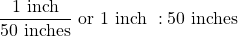Self: At this point the 1 inch works as we’re looking for inches per foot but the 50 inches has to be put into feet. To do this we’ll take the fifty and divide it by 12. This will get us into feet.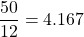Me: Okay. I’m seeing where you’re going with this. I’m picking up what you’re putting down. Now we have to put 4.167 into a ratio.

Self: Yup.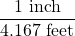Me: I see we’re not quite there yet.

Self: Correct. Remember the grade is expressed in inches per foot therefore we must get the 4.167 feet back to one foot. The easiest way to do this is to divide both parts of the ratio by 4.167. We’ll then end up with one foot.

Start with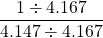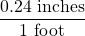Self: We end up with 0.24 inches per foot. This is basically one quarter on an inch per foot.I know I’ve said this before but I’m going to say it again. Learning the concepts surrounding math is far more important than memorization. In the end you already have an idea what the solution is going to be. You just have to put all the pieces of the puzzle together. You don’t need to memorize each piece of the puzzle but what you do instead is generate each piece of the puzzle as you go. This is accomplished by taking some time to understand what the question is asking and beginning to draw out the route you take to get there. Keep that in mind as you go through this book.# Inches Per Foot to Percent and Back

We’ve already gone through a lot of the initial math regarding inches per foot in the last example. We’ll work with one quarter of an inch per foot and just skip a bit of the math that we already did before. Remember that the whole idea is that we have to get the inches per foot to work for us. That means we have to get both of the units to be the same. In this case we will once again go with inches.

Start with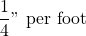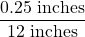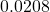What we have in the end is 0.0208. What does that do for us? There are actually two parts to this answer.

If we were trying to find the total fall on an object the number 0.0208 is what we would use in the formula. If we wanted to find out what 0.0208 is as a percent then we have to change the number. How we do this is by multiplying by 100.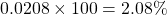In the end of the day we have a 2.08 percent grade.Now let’s do the reverse. We’ll go from a percent back into inches per foot.

This time we’ll start with 3 percent and work our way back to inches per foot.

Start with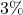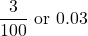We’ll use the ratio 3 over one hundred as it’s easier to work with. Remember that in the case of a ratio there are no units. What we are saying with a 3 percent grade is that for every 100 units that the object goes horizontally it falls 3 units vertically.

Now if we are changing the grade to inches per foot we could then say that for every 100 feet that the object goes horizontally it falls 3 feet vertically.

Let’s start to look at this from a mathematical perspective. If the object falls 3 feet we could then say it falls 36 inches.

It looks as though we are starting to get somewhere. We are starting to get to that “inches per foot” grade we are looking for. The only issue we have to solve is the fact that at the moment we are dealing with one hundred feet. We have to get that down to one foot. In this case we take the one hundred and divide it by one hundred. That leaves us with one. We also do this to the 36 inches to keep things mathematically consistent.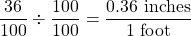Now we’re really getting somewhere. I think we’re almost there. The only thing left to do is change the 0.36 inches into some type of fraction of an inch. We can put it into quarters of an inch, eighths of an inch, sixteenths of an inch or even thirty seconds of an inch. For our purposes let’s go with eighths of an inch.

By the way if you don’t quite remember how to go from decimals of an inch to fractions of an inch you can always go back to the first volume of Math for Trades and do a little refresher. If you do remember check out the answer just below.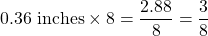In the end a grade of 3 percent changed to inches per foot works out to be 3/8″/ft.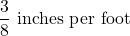Try a few practice questions. Check the videos answers to see how you did.

# Practice Questions

Question 2

A piece of pipe grades down at a rate of 3/8″ per foot. What would that grade be as a ratio over 100?

Question 3The piping for a fire sprinkler system is run at a grade of 7:198. What is this grade expressed as a percent?

Note: My apologies to all those sprinkler fitters out there. This is not a realistic grade for the pipe you run. Take one for the purpose of math.